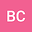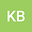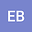Extracting information from ocean color
•••• +1## Abstract

Products derived from remote sensing reflectances ($R_{rs}(\lambda)$), e.g. chlorophyll, phytoplankton carbon, euphotic depth, or particle size, are widely used in oceanography. Problematically, $R_{rs}(\lambda)$ may have fewer degrees of freedom (DoF) than measured wavebands or derived products. A global sea surface hyperspectral $R_{rs}(\lambda)$ dataset has DoF=4. MODIS-like multispectral equivalent data also have DoF=4, while their SeaWiFS equivalent has DoF=3. Both multispectral-equivalent datasets predict individual hyperspectral wavelengths’ $R_{rs}(\lambda)$ within nominal uncertainties. Remotely sensed climatological multispectral $R_{rs}(\lambda)$ have DoF=2, as information is lost by atmospheric correction, shifting to larger spatiotemporal scales, and/or more open-ocean measurements, but suites of $R_{rs}(\lambda)$-derived products have DoF=1. These results suggest that remote sensing products based on existing satellites’ $R_{rs}(\lambda)$ are not independent and should not be treated as such, that existing $R_{rs}(\lambda)$ measurements hold unutilized information, and that future multi- or especially hyper-spectral algorithms must rigorously consider correlations between $R_{rs}(\lambda)$ wavebands.